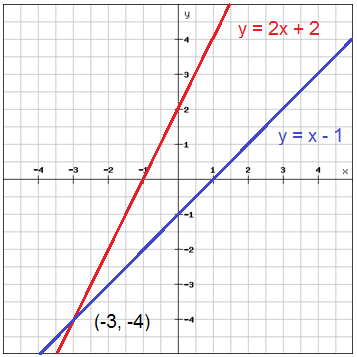# Solve systems of equations by graphing

A system of linear equations contains two or more equations e.g. y=0.5x+2 and y=x-2. The solution of such a system is the ordered pair that is a solution to both equations. To solve a system of linear equations graphically we graph both equations in the same coordinate system. The solution to the system will be in the point where the two lines intersect.

Example

$\left\{\begin{matrix} y=2x+2\\ y=x-1\: \: \: \end{matrix}\right.$

Graph the equations in a coordinate planeThe two lines intersect in (-3, -4) which is the solution to this system of equations.

## Video lesson

Find the solution of two equations by graphing